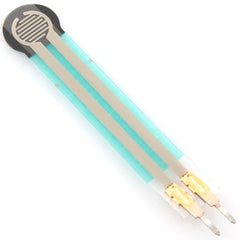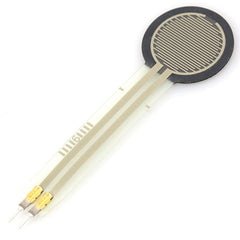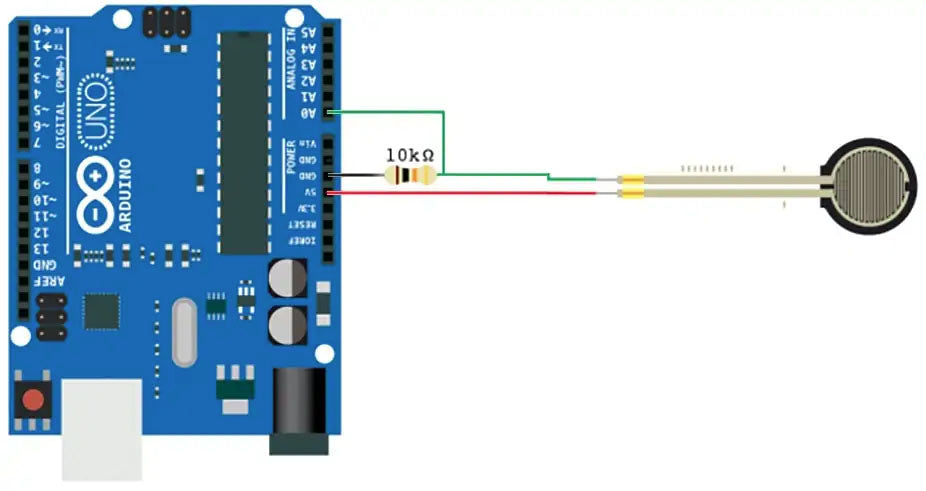# Using a Force Sensitive Resistor (FSR) with Arduino

## So.... What is an FSR / Force Sensitive Resistor?You know when people say the clue is in the name… Well, sometimes it really is. A Force Sensitive Resistor, or FSR as we’ll call it, is a resistor that changes its resistance with force. When you press, stand, or hit it, its resistance changes.FSRs come in a wide variety of sizes, so are can be used for a huge array of projects. Maybe an electronic whoopee cushion??? Combine with a speaker, amp, and a microprocessor loaded with some “popping off” sounds connected to an FSR under a chair leg! Viola!While these devices are easy to use, versatile and pliable, they can be pricey depending on the size that you need. They also don’t have a huge amount of accuracy, so if you are looking to acquire accurate measurements, you really want to look at load cells!

### Connecting an FSR to Arduino:The FSR changes its resistance with force. It ranges from near-infinite with no force applied, to under 300Ω when fulled loaded. Using the analog inputs from a MCU such as an Arduino you can measure that change.

When connecting the Arduino you’ll need to add a resistor that we can use for a reference. We suggest using a 10K resistor. This circuit is called a voltage divider and divides the 5v between the FSR and the resistor.Understanding the Output:

The Arduino analogue input is essentially a voltage meter and at 5V (Maximum value for Arduino Uno) it will read 1023, and at 0v it will read 0.

Knowing this you can measure how much voltage is on your FSR using the analogRead. This will in turn tell you how much force is being applied to your FSR.

The amount of that 5V that each part gets is proportional to its resistance. So if the FSR and the resistor have the same resistance, the 5V is split equally (2.5V) to each part. This would give you an analog reading of 512.

But if the FSR is pressed on pretty hard, reading only 1K of resistance, the 10K resistor is going to soak up 10 times as much of that 5V. So the FSR would only get .45V. This would give you an analog reading of 92.

And if something is barely pressing on it, the FSR maybe 40K of resistance, so the FSR will soak up 4 times as much of that 5V as the 10K resistor. So the FSR would get 4V. This would give you an analog reading of 819.

### Voltage Divider Equation:• In this equation, the output voltage increases with increasing force.
• If RFSR and RM are swapped, the output swing will decrease with increasing force.